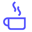a concise shot of dev knowledge
Become a Contributor
Concise shots of dev knowledge

RELATED TAGS

sampling
algorithms

# What is reservoir sampling?Edpresso Team

Reservoir sampling is a randomized algorithm that is used to select $k$ out of $n$ samples; $n$ is usually very large or unknown. For example, reservoir sampling can be used to obtain a sample of size $k$ from a population of people with brown hair. This algorithm takes $O(n)$ to select $k$ elements with uniform probability.Reservoir sampling is used to randomly take k out n samples. Here k = 4.

## Algorithm

1. Copy the first $k$ elements from the input array to the output array.

2. Iterate from $k$ to $n-1$ (both inclusive). In each iteration $j$:

2.1 Generate a random number $num$ from 0 to $j$.

2.2 If $num$ is less than $k$, replace the element at index $num$ in the output array with the item at index $j$ in the input array.

## Code

// Including dependancies
#include <iostream>
#include <stdlib.h>
#include <time.h>
using namespace std;

int main() {
// Defining the parameters
int k = 4;
int n = 8;
// The array to be sampled
int input[] = {1, 7, 4, 8, 2, 6, 5, 9};
int output[k];
// Getting a random seed everytime
srand (time(NULL));
int i;
// Initializing the output array to the first k elements
// of the input array.
for(i = 0; i < k; i++){
output[i] = input[i];
}
int j;
// Iterating over k to n-1
for(j = i; j < n; j++){
// Generating a random number from 0 to j
int index = rand() % (j + 1);
// Replacing an element in the  output with an element
// in the input if the randomly generated number is less
// than k.
if(index < k){
output[index] = input[j];
}
}
cout<<"Input array:"<<endl;
for( i = 0; i < n; i++){
cout<<input[i]<<" ";
}
cout<<endl;
cout<<"Output array:"<<endl;
for(i = 0; i < k; i++){
cout<<output[i]<<" ";
}
cout<<endl;
return 0;
}

RELATED TAGS

sampling
algorithms
RELATED COURSES

View all Courses

Keep Exploring

Learn in-demand tech skills in half the time